# Create a line plot using pandas DataFrame (`pandas.DataFrame.plot.line`)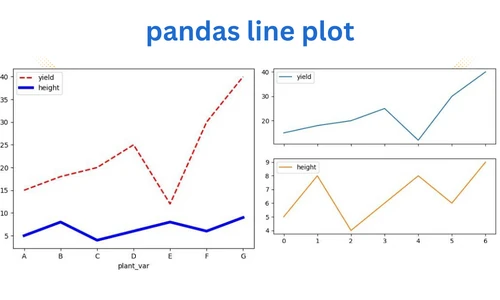The line plots are useful to study the relationship between the variables by connecting the data points as line. The lines can be straight or curved.

In Python, line plots are typically created from numerical arrays using the `pyplot.plot()` function available in the matplotlib package. While you are working on the DataFrame, pandas plotting features can be handy for creating line plots.

This tutorial will show you how to create a line plot directly from a pandas DataFrame columns using a `plot.line()` function. The `plot.line()` function takes the following arguments for creating a line plot,

• x: column name for plotting data on X-axis
• y: column name for plotting data on Y-axis
• color: the color of the points (RGB or RGBA code). It can take a single scalar value or multiple values
Now, let’s create a line plot using pandas `plot.line()`

## Import the pandas library

Import the pandas library

``````import pandas as pd
``````

## Create a basic pandas line plot

As a first step, we will create a basic line plot (with default settings) using pandas Dataframe. To create this line plot, we will use a pandas DataFrame containing the types of plant varieties, their yields, and their heights.

``````# create a pandas DataFrame
df = pd.DataFrame({'plant_var':['A', 'B', 'C', 'D', 'E', 'F', 'G'], 'yield':[15, 18, 20, 25, 12, 30, 40],
'height':[5, 8, 4, 6, 8, 6, 9] })

# view first two rows of dataset
# output
plant_var  yield  height
0         A     15       5
1         B     18       8

# create a line plot
df.plot.line() # same as df.plot()
plt.show()
``````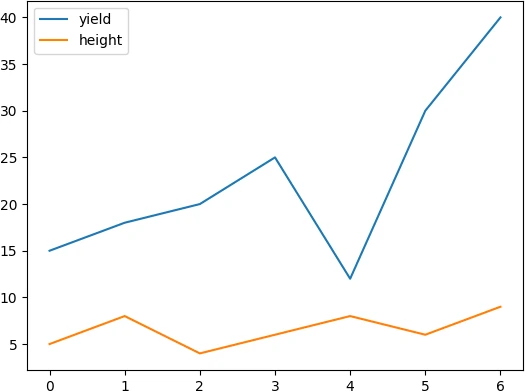To add the labels to X-axis, you can make `plant_var` column as index and then create a line plot,

``````# set first column as index
df = df.set_index('plant_var')

# view first two rows of dataset
yield  height
plant_var
A             15       5
B             18       8

# create a line plot
df.plot.line()
plt.show()
``````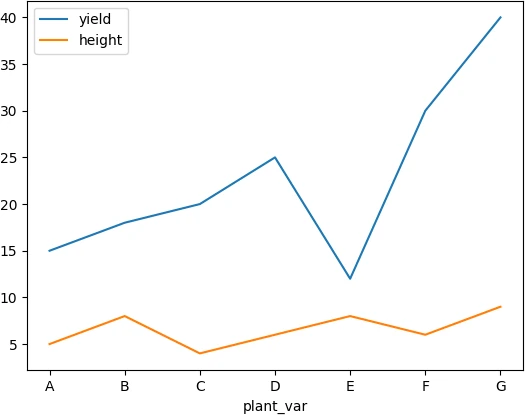## Change color, style, linewidth, and transparency

Change the colors (`color`), style, transparency (`alpha`), and linewidth of the lines in pandas line plot,

``````df.plot.line(color = ['r', 'b'], linestyle = 'dashed', alpha = 0.5, linewidth = 2)
plt.show()
``````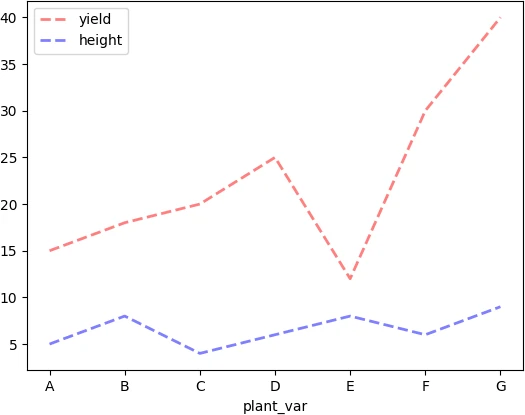## Add marker and change figure size

You can use the `marker` parameter to add the marker to the lines. Similarly, the `figsize(width, height)` parameter can be used for changing the figure size of the pandas line plot.

``````df.plot.line(color = ['r', 'b'], marker = 'o', linestyle = 'dashed', linewidth = 2, figsize = (6, 5) )
plt.show()
``````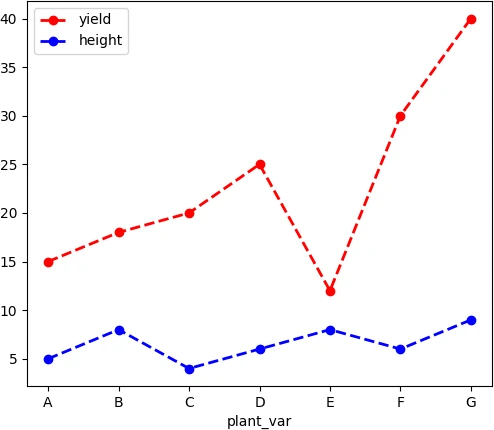## pandas multiple lines plot with customized options

You can plot multiples lines in a pandas line plot with customized parameters for each line. Here, you need to use `plt.subplots()` to get a tuple of figure (`fig`) and axis (`ax`) objects. The axis object is useful for customizing each line (e.g. for line style, color, width, etc.) in same line plot. Here, we need to specify the X and Y-axis names as column names from DataFrame.

``````# create DataFrame
df = pd.DataFrame({'plant_var':['A', 'B', 'C', 'D', 'E', 'F', 'G'], 'yield':[15, 18, 20, 25, 12, 30, 40],
'height':[5, 8, 4, 6, 8, 6, 9] })

# line plot
fig, ax = plt.subplots()
df.plot.line(ax = ax, x = 'plant_var',y = 'yield', color = 'r', linewidth = 2, linestyle ='dashed')
df.plot.line(ax = ax, x ='plant_var', y = 'height', color = 'b', linewidth= 4, linestyle = 'solid')
plt.show()
``````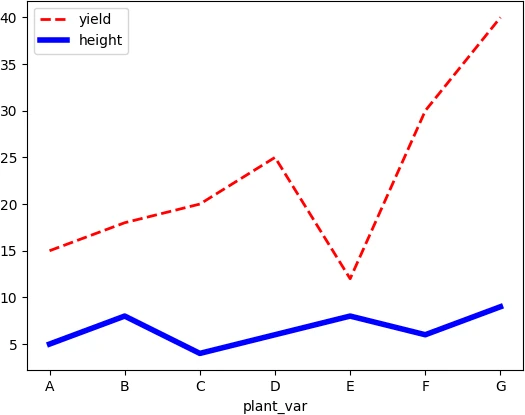If you want to create two subplots, add the `subplots=True` parameter to the `line()` function,

``````# create two subplots
fig, ax = plt.subplots()
df.plot.line(subplots=True)
plt.show()
``````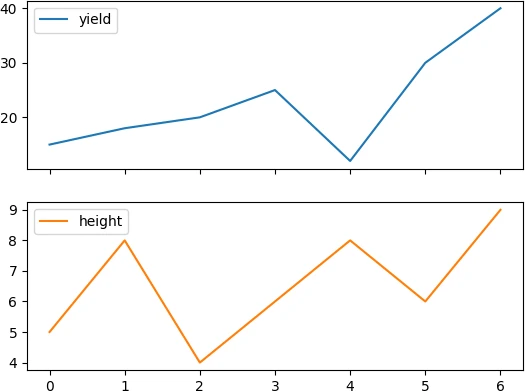## pandas multiple lines plot for categorical column

You can plot multiple lines based on the categorical column in pandas Dataframe. here, you will use the pandas `groupby()` function to create a line plot. To create this pandas groupby line plot, we will create a time series dataset as pandas DataFrame containing the year of planting, types of plant varieties, and their yields.

``````# create DataFrame
df = pd.DataFrame({'year':['2018', '2019', '2020', '2021', '2022', '2018', '2019', '2020', '2021', '2022'],
'plant_var':5*['A'] + 5*['B'],
'yield': [15, 18, 20, 25, 22, 25, 20, 28, 24, 30] })

# view first two rows of DataFrame
# output
year plant_var  yield
0  2018         A     15
1  2019         A     18

# pandas groupby line plot (plant_var column)
df = df.set_index('year')
df.groupby('plant_var')['yield'].plot(legend=True, marker = 'o', linewidth = 2)
plt.show()
``````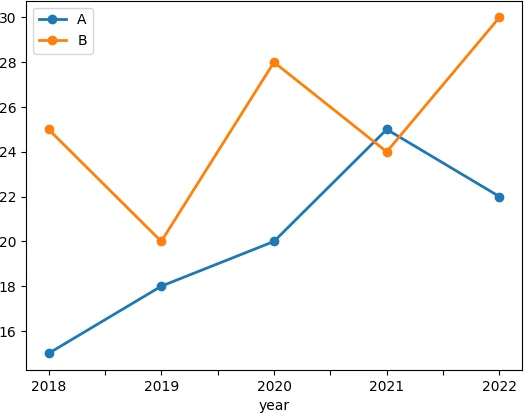If you want to create two subplots based on the categorical column, you will first need to reshape the DataFrame with a categorical column and split that into separate columns. You can use the pandas `pivot()` function to reshape the DataFrame.

``````df = pd.DataFrame({'year':['2018', '2019', '2020', '2021', '2022', '2018', '2019', '2020', '2021', '2022'],
'plant_var':5*['A'] + 5*['B'],
'yield': [15, 18, 20, 25, 22, 25, 20, 28, 24, 30] })

# first reshape the DataFrame
df_reshape = df.pivot(index='year', columns='plant_var', values='yield')
# view DataFrame
df_reshape
# output
plant_var   A   B
year
2018       15  25
2019       18  20
2020       20  28
2021       25  24
2022       22  30

# create a grouped line plots as subplots
df_reshape.plot.line(subplots=True)
plt.show()
``````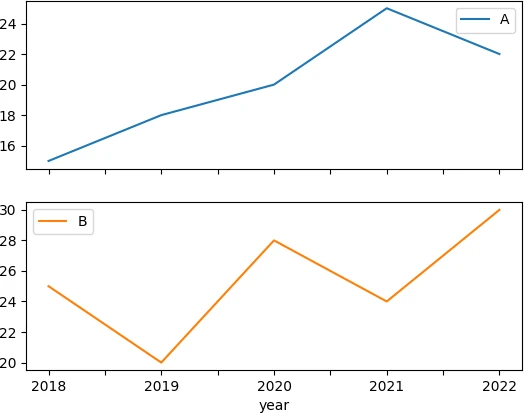In summary, you learned how to create a pandas line plot on DataFrame using `plot.line()` function.